# How to Add & Subtract Two Fractions with Like Denominators

Instructor: Heather Jenkins

Heather has a bachelor's degree in elementary education and a master's degree in special education. She was a public school teacher and administrator for 11 years.

Although fractions may look different from whole numbers, we can still add and subtract them. In this lesson, you will learn how to add and subtract two fractions with like denominators.

## Adding Fractions with Like Denominators

If you have ever helped with cooking, had your height measured, or tried on a pair of shoes, you have most likely encountered fractions. Fractions are useful in our everyday lives when we need to express a number that is not quite one whole or when we want to show a part of a set. Sometimes we may have situations where we have to add or subtract fractions as well. Let's take a look at how to add and subtract fractions with like denominators.

When adding or subtracting fractions, the first step is to check that the denominators of the fractions are the same. The denominator is the number underneath the fraction bar, which represents the total number of parts in one whole. For example, in this problem, the denominators are both 8.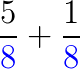Since the denominators are the same, the denominator of the answer will also be the same.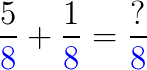Next, add the numerators together. The numerator is the number on top of the fraction bar. For example, in this problem, the two numerators are 5 and 1. When you add these together, this will give you the numerator of your answer. So the answer is 6/8.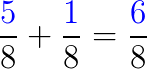## Subtracting Fractions with Like Denominators

Check to make sure the denominators are the same. For example, in this problem, the denominators are both 9.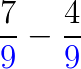Since the denominators are the same, the denominator of the answer will also be the same.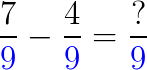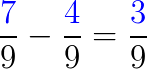To unlock this lesson you must be a Study.com Member.

### Register to view this lesson

Are you a student or a teacher?

#### See for yourself why 30 million people use Study.com

##### Become a Study.com member and start learning now.
Back
What teachers are saying about Study.com

### Earning College Credit

Did you know… We have over 200 college courses that prepare you to earn credit by exam that is accepted by over 1,500 colleges and universities. You can test out of the first two years of college and save thousands off your degree. Anyone can earn credit-by-exam regardless of age or education level.